# Deep Learning笔记1

## 逻辑回归与神经网络

Posted by mingjie on March 14, 2018

### 逻辑回归

#### Binary Classification

Binary Classification指的是输入一堆东西，最后给出一个只有两个结果（0或者1）的输出的分类过程。一个典型而符合惯例的例子是判断某张图片上有没有猫，也就是将图片的红绿蓝像素值矩阵作为输入，是猫(1)以及不是猫(0)作为输出。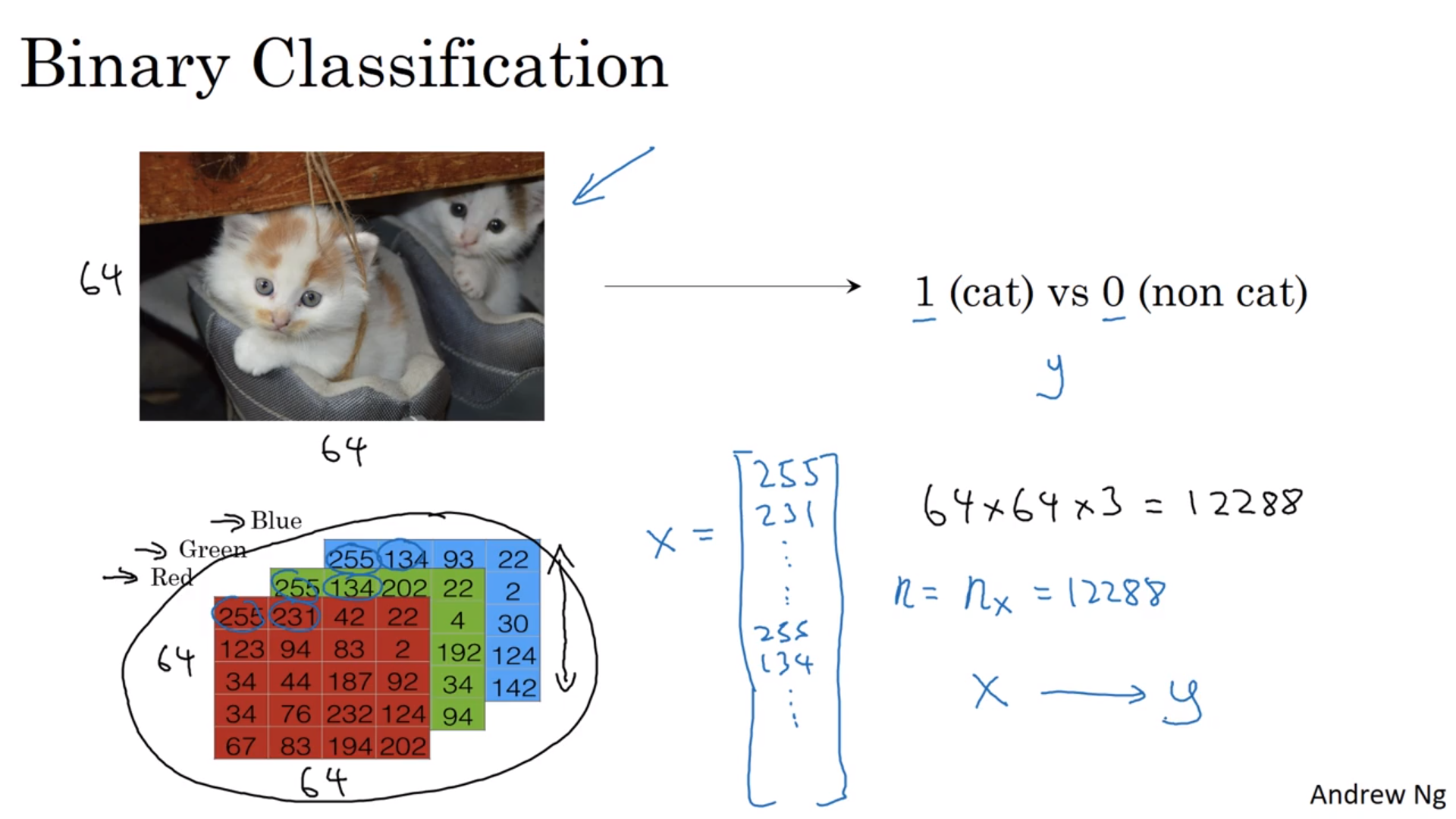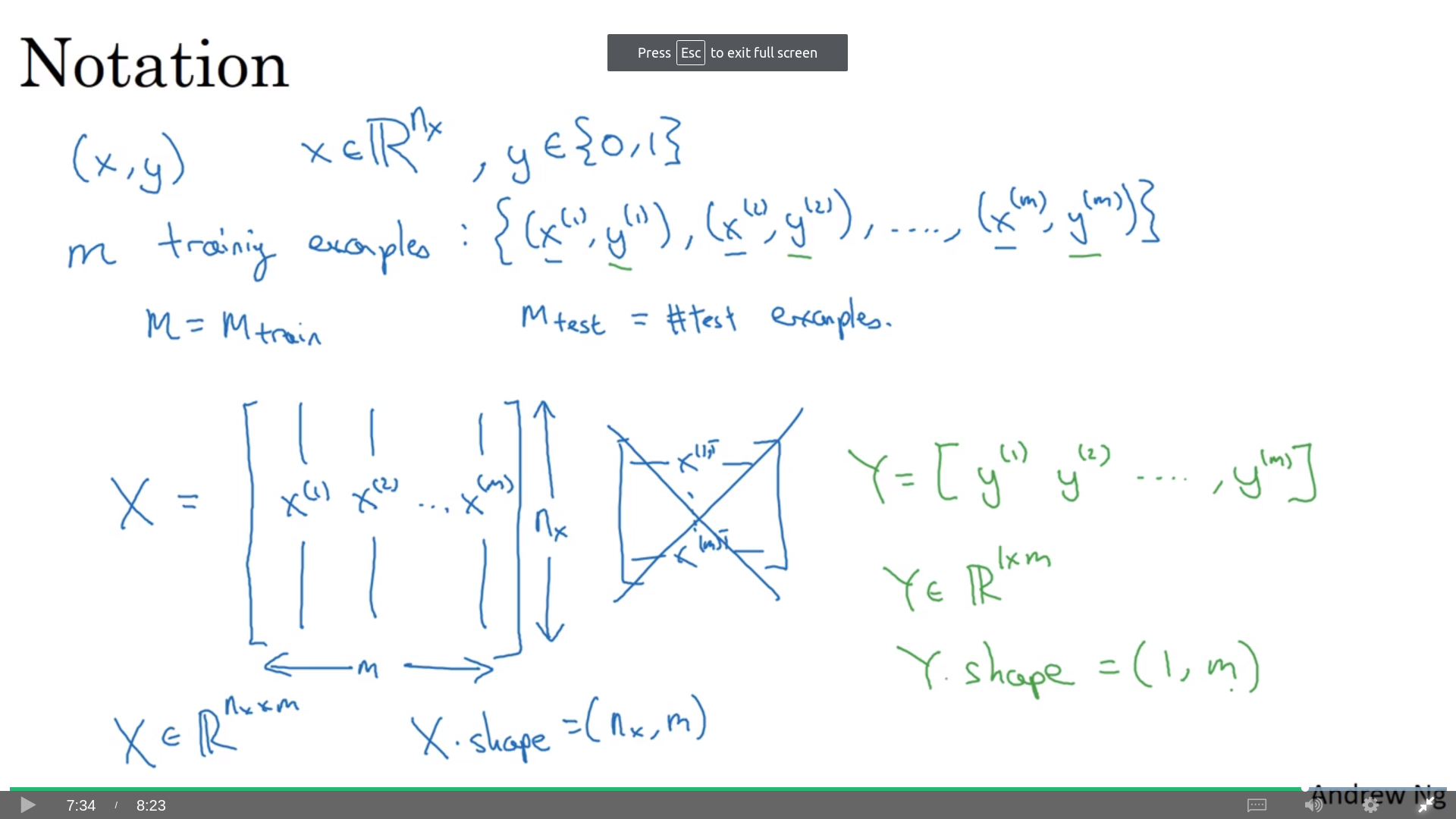#### 逻辑回归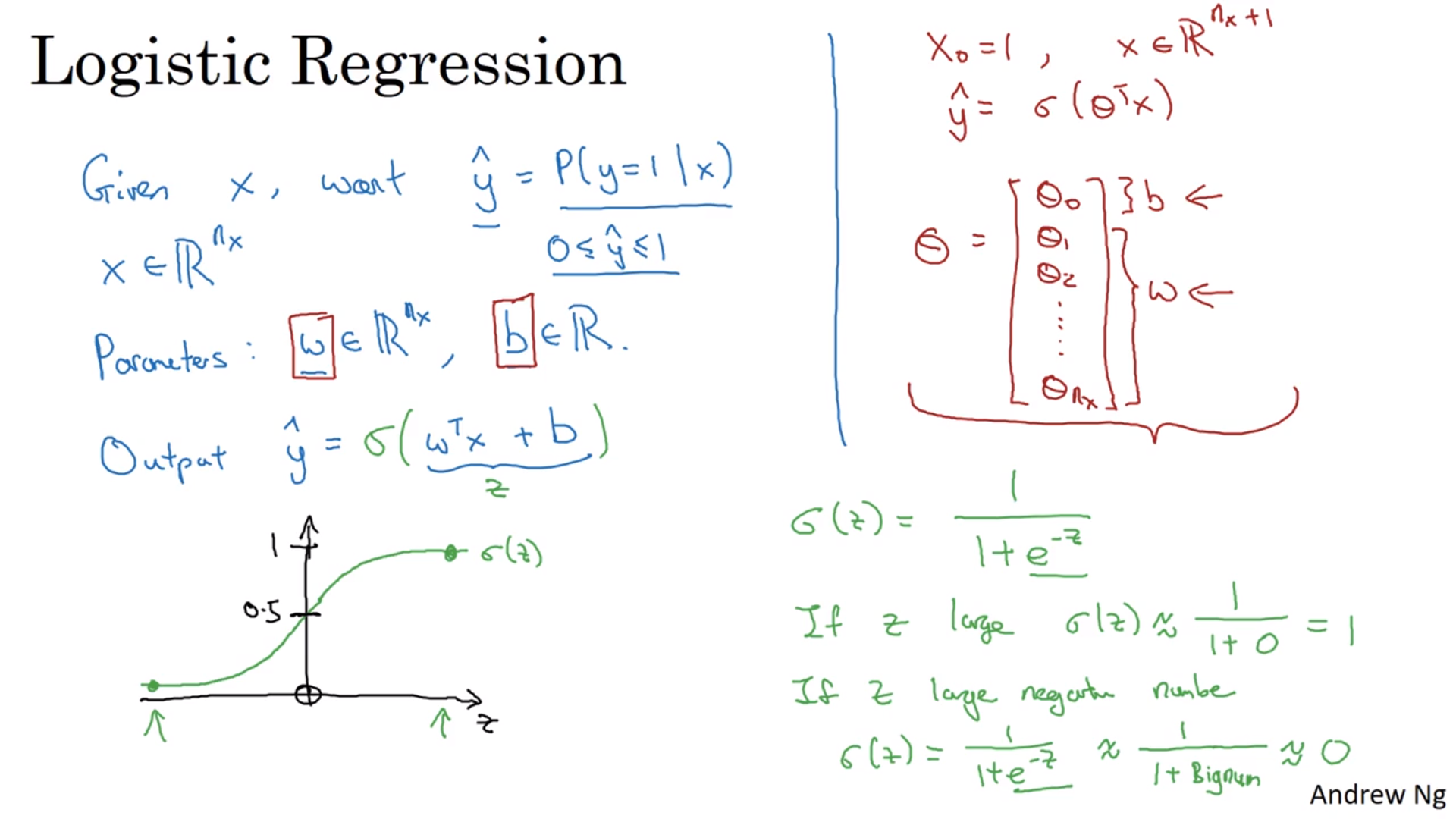#### 代价/损失函数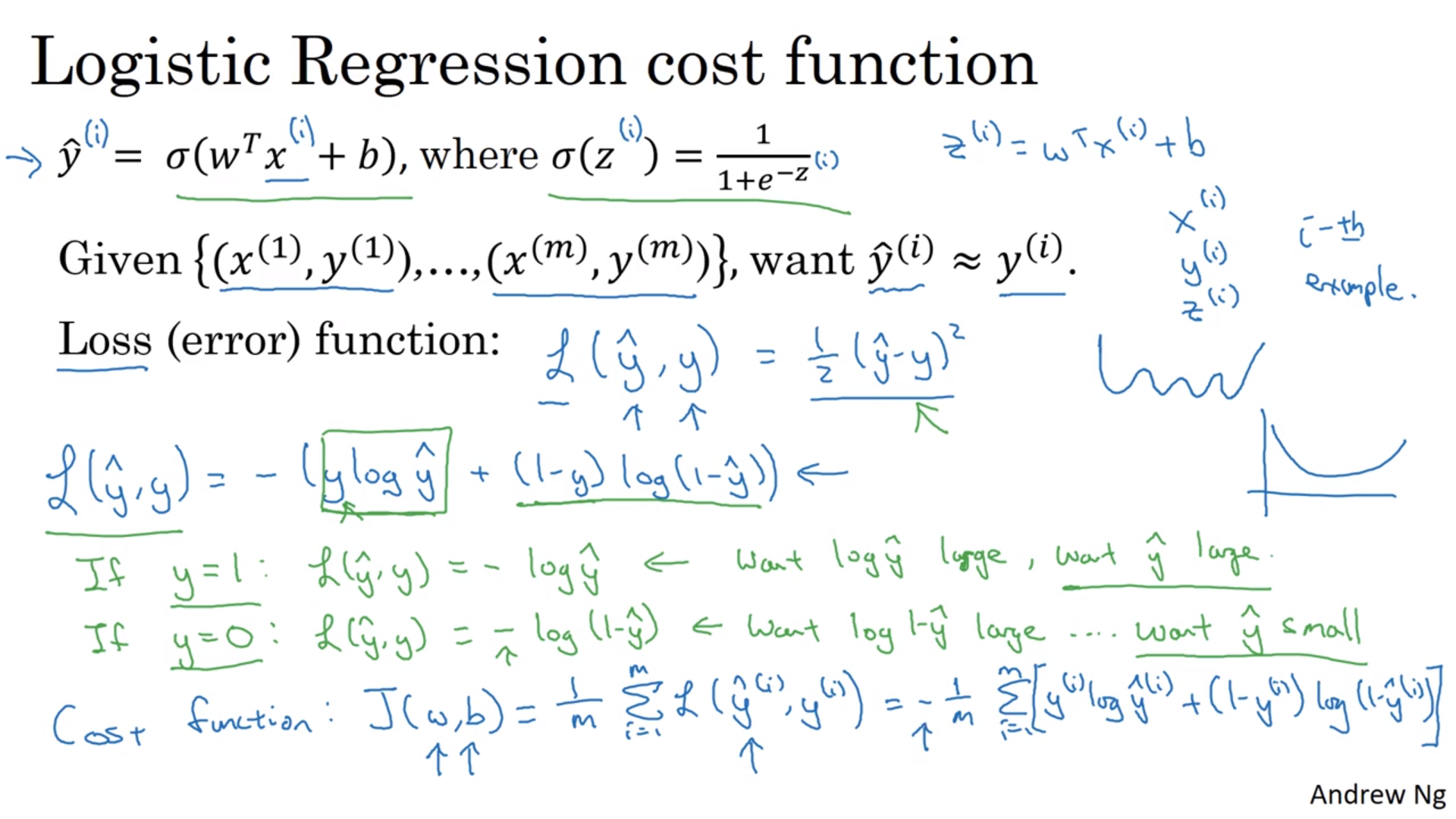#### 如何训练参数？

$\alpha$被称为学习速率，是人选的；而后面的俩偏导数是需要计算的，在python中会被命名为dw, db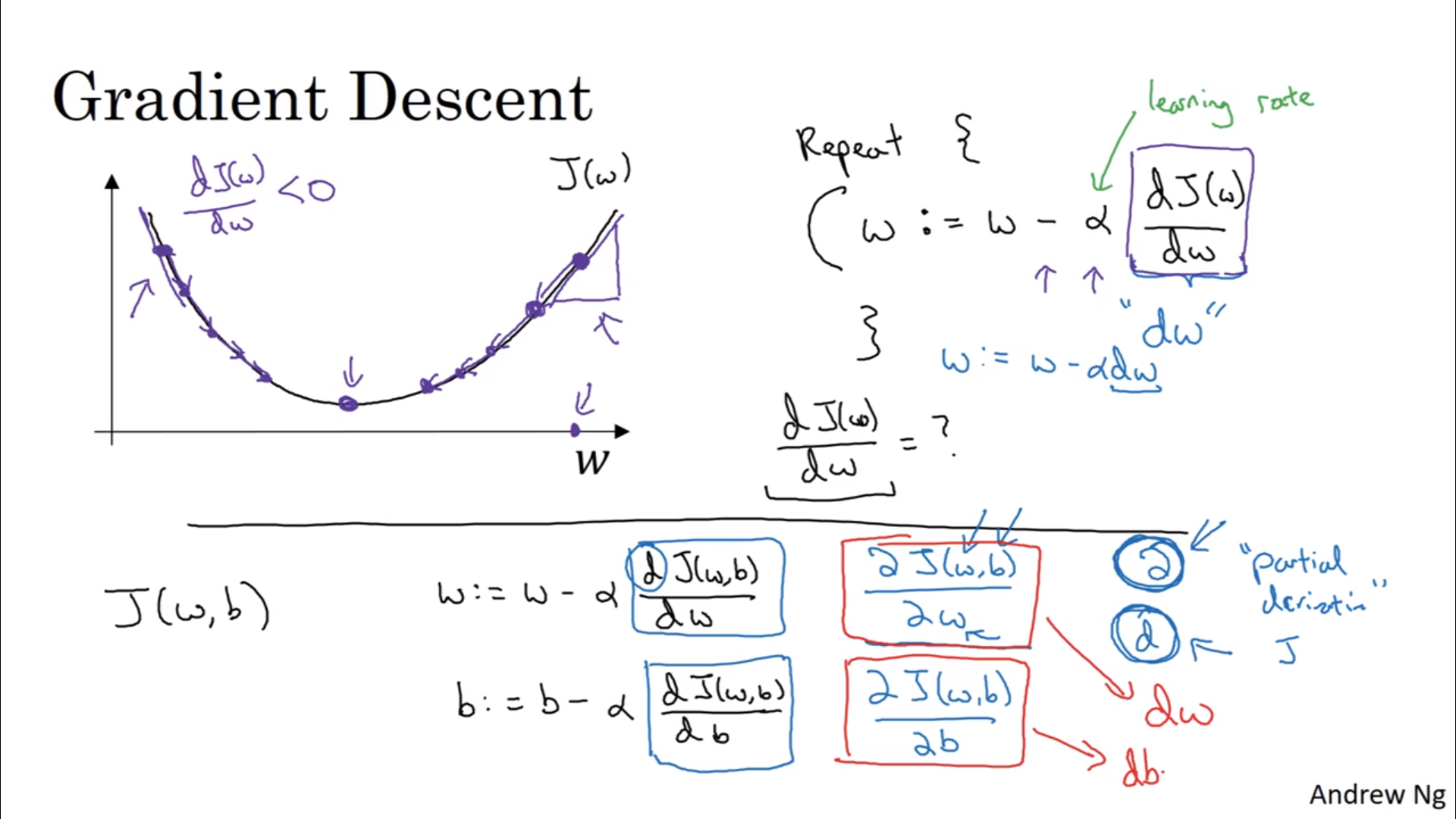#### 正反向传递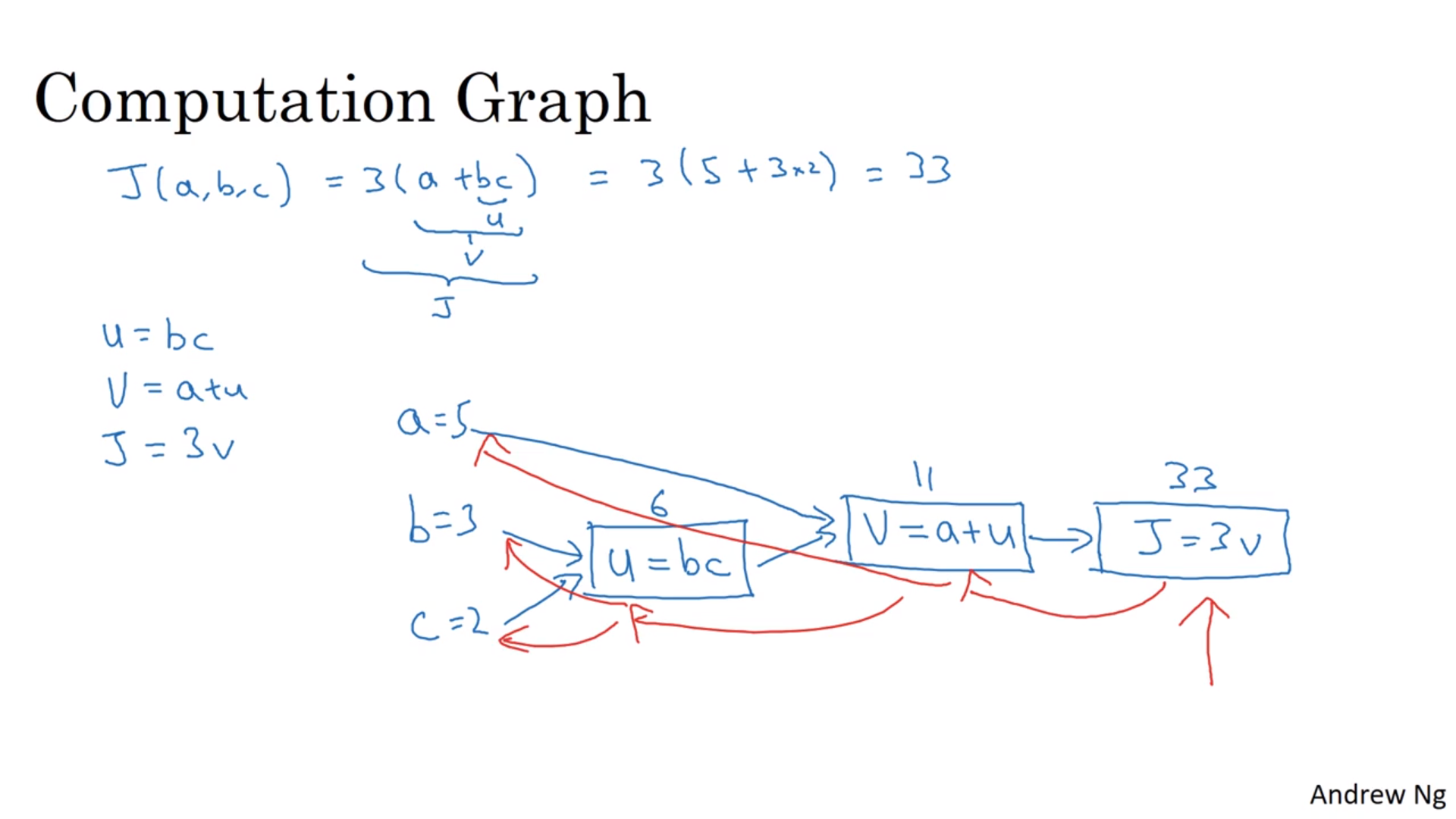#### 导数的具体计算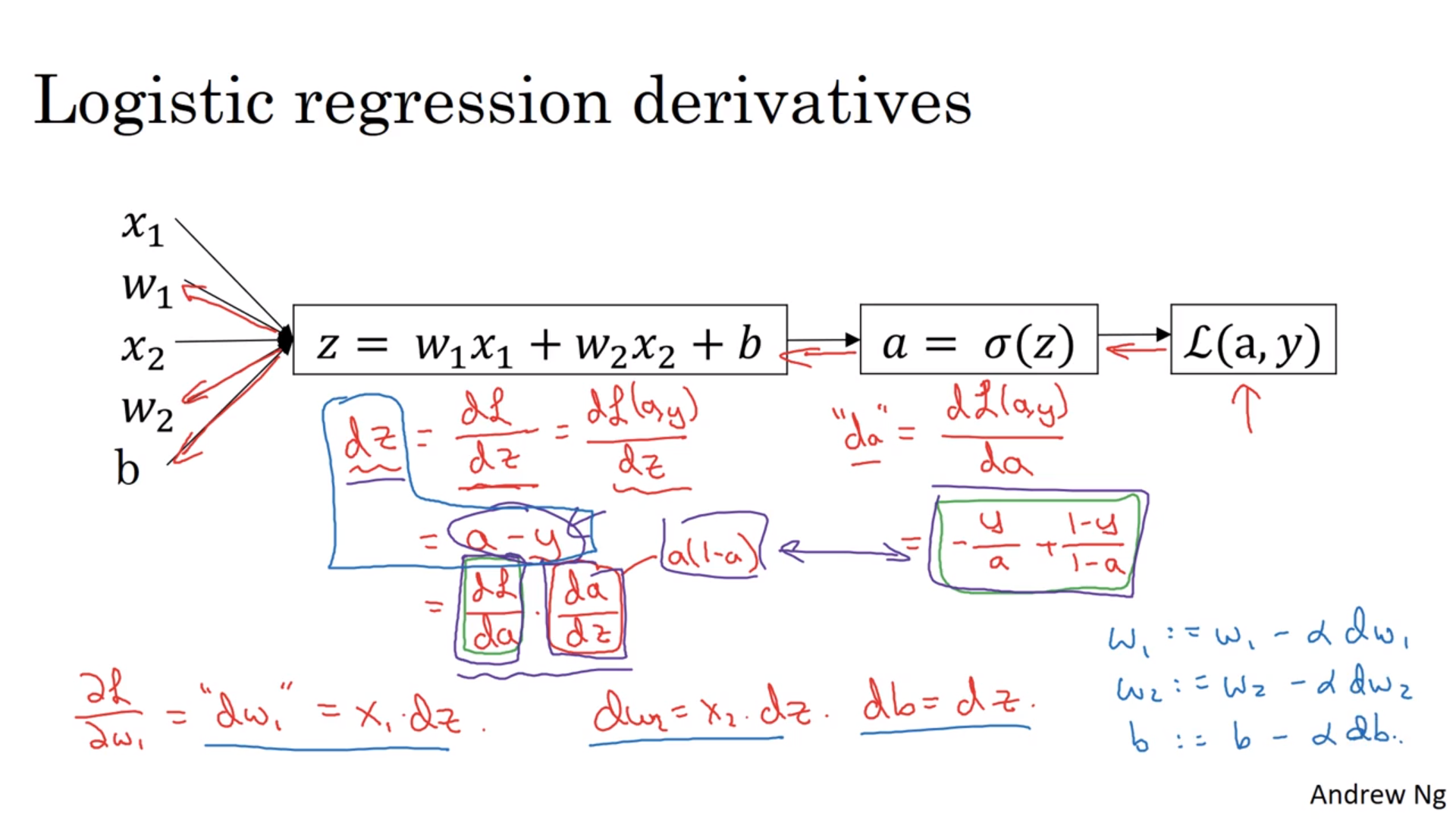# 设置初值
J = 0; dw1 = 0; ...; db = 0
for i in np.arange(1,m+1):
# Forward propagation
z[i] = w.T * x[i] + b
a[i] = sigmod(z[i])
J += L(a[i], y[i])
# Backward propagation
dz[i] = a[i] - y[i]
dw1 += x1[i]dz[i]
...
db += dz[i]
J /= m; dw1 /= m; ...; db /= m
w1 = w1 - alpha * dw1
...
b = b - alpha * db


#### 向量化

w是一个列向量的时候，比如要算z[i] = w.T * x[i] + b的时候，可以这么做：np.dot(w,x)+b。高级一点的话直接和矩阵相乘：np.dot(w,X)+bdz[i] = a[i] - y[i]可以转为dZ = A - Ydw1 += x1[i]dz[i] -> dW = 1/m * X*dZ.Tdb += dz[i] -> 1/m * np.sum(dZ)。还要注意的一点是在reshape的时候最好把行列都写全。

### 浅神经网络

,代表神经网络的层数；(i)代表某一个训练样本（都是上标）。下标不带任何括号，表示某一层中的第几个节点。当有两层的时候，刚开始的输入是$x$，但是之后的输入会变成了$a^{[i]}$这样。

#### 神经网络的命名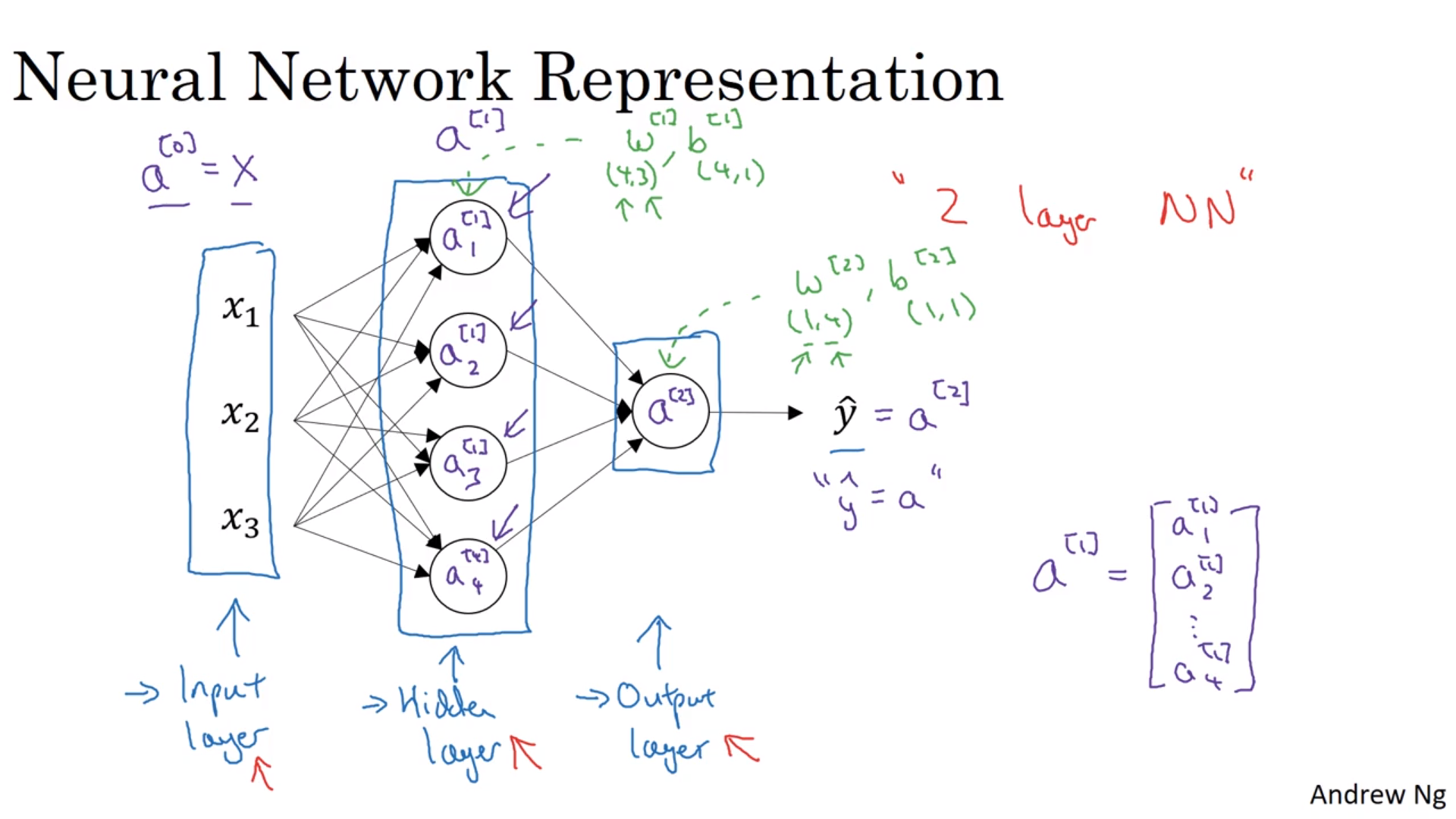$W^{[i]}$是一个$n^{[i]}$（本层节点数）行$n^{[i-1]}$（上层节点数）列的矩阵，也就是负责将上层节点的结果转成本层节点的结果。$b^{[i]}$自然是$n^{[i]}$行1列的向量了。最后再将结果各自丢进sigmod函数里面，不说了。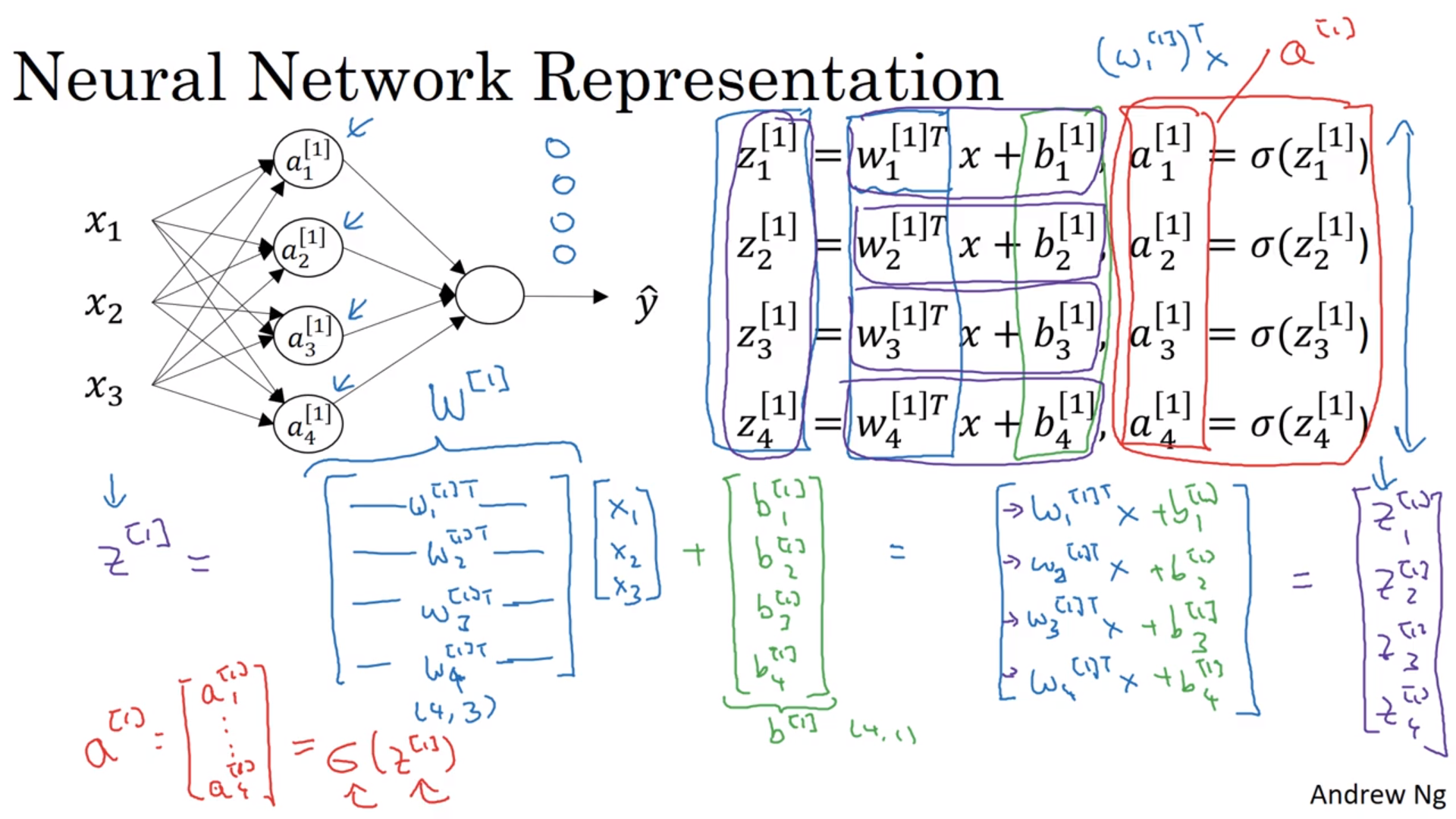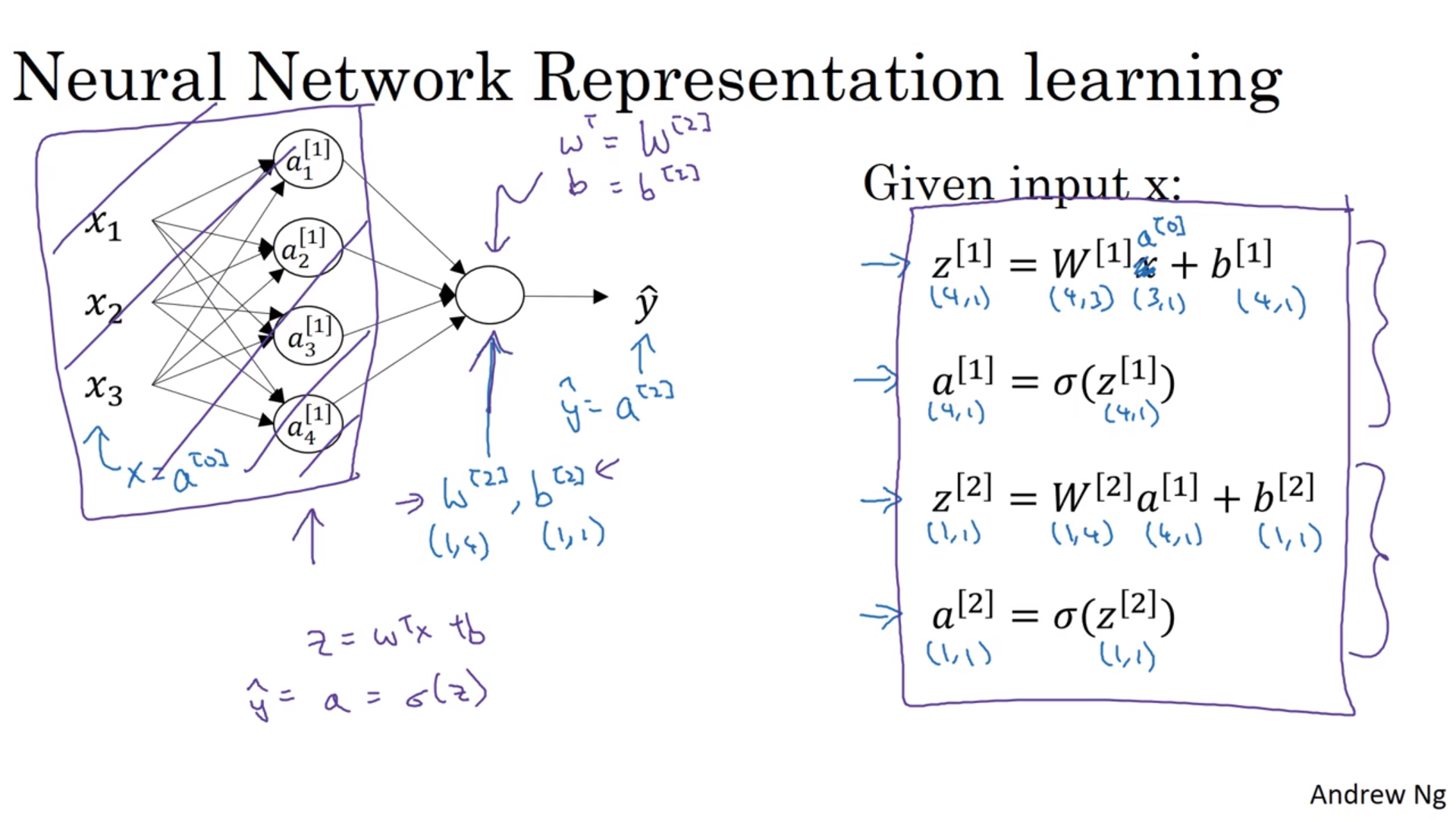#### 对于整个训练样本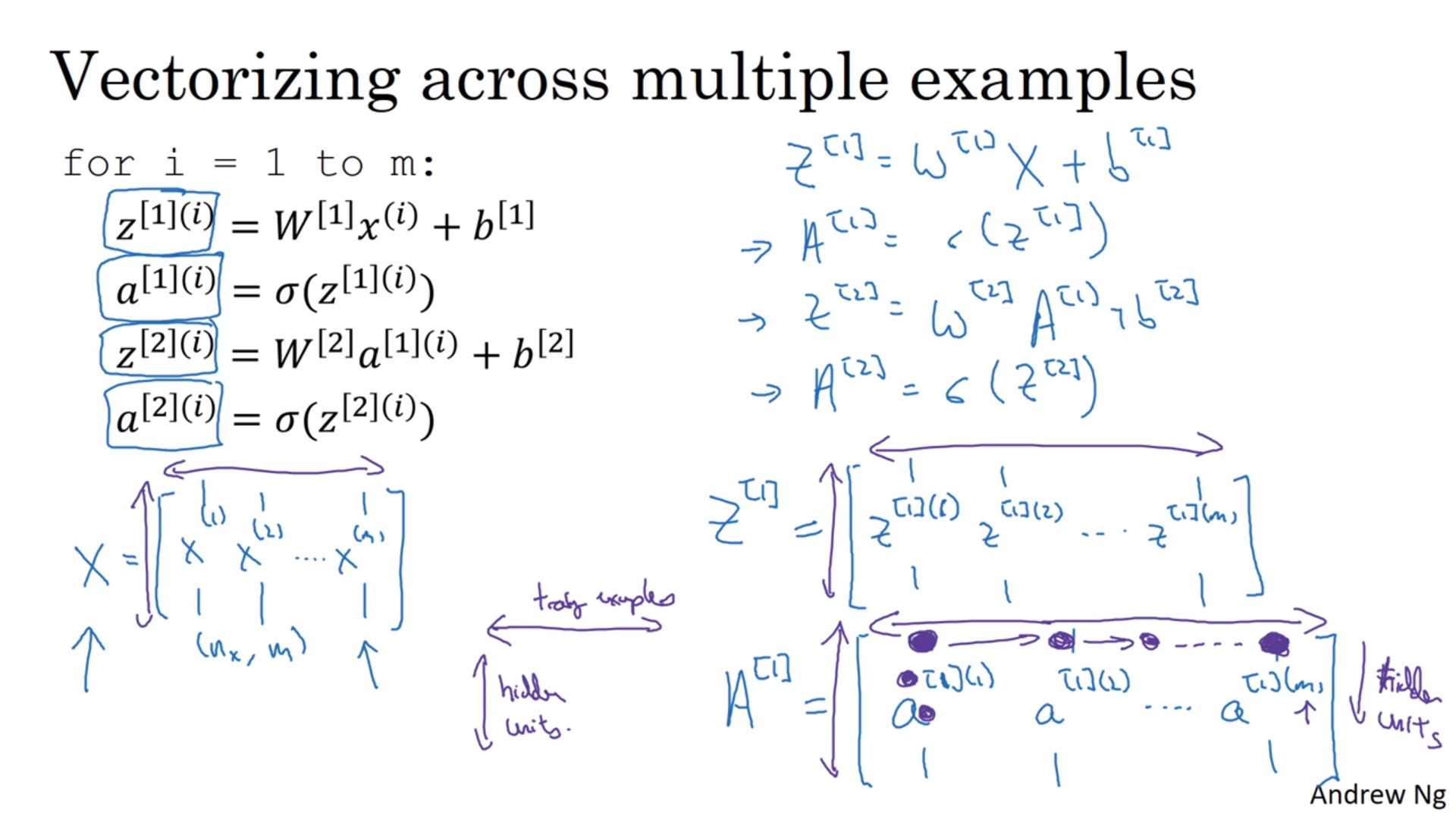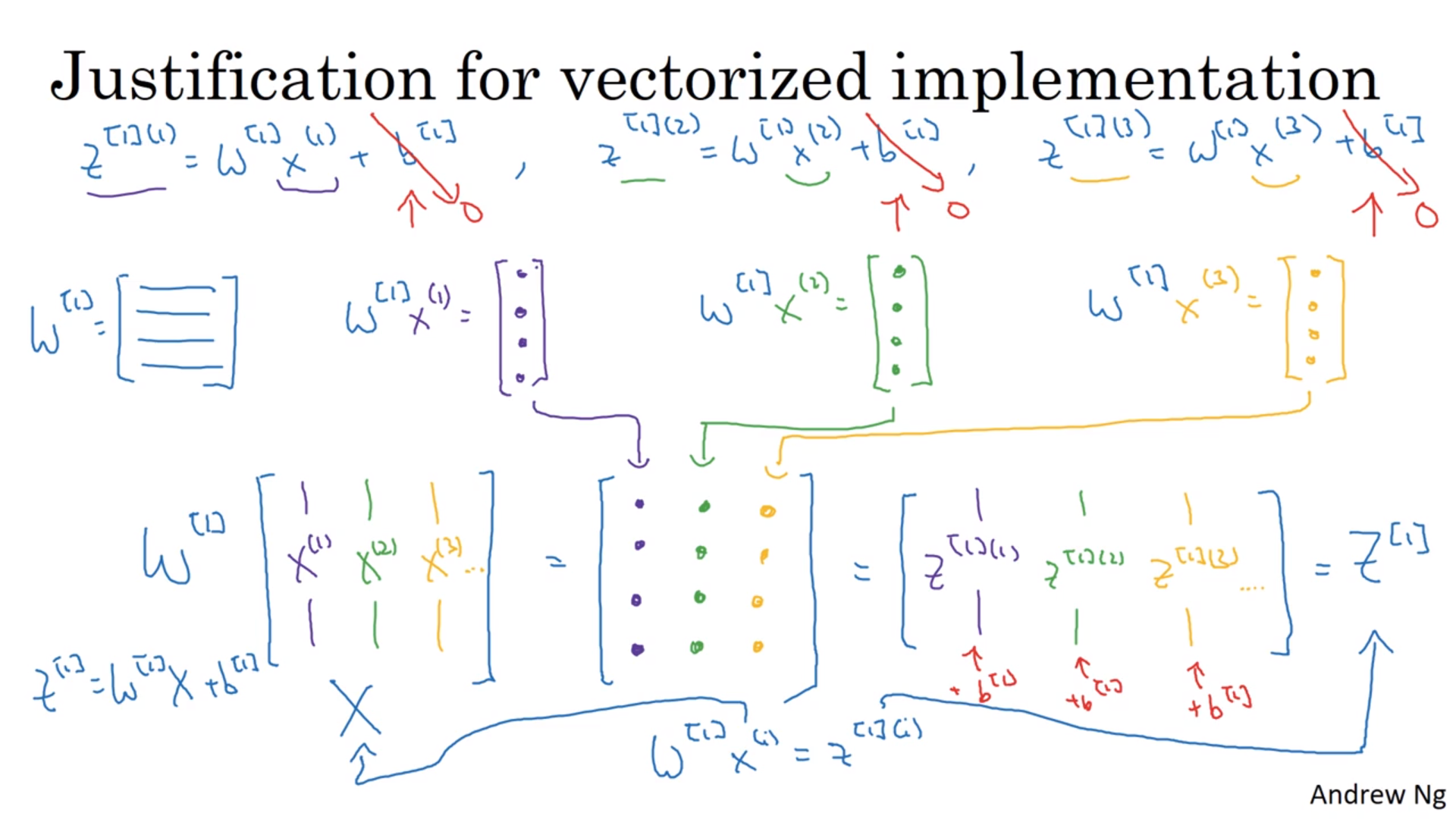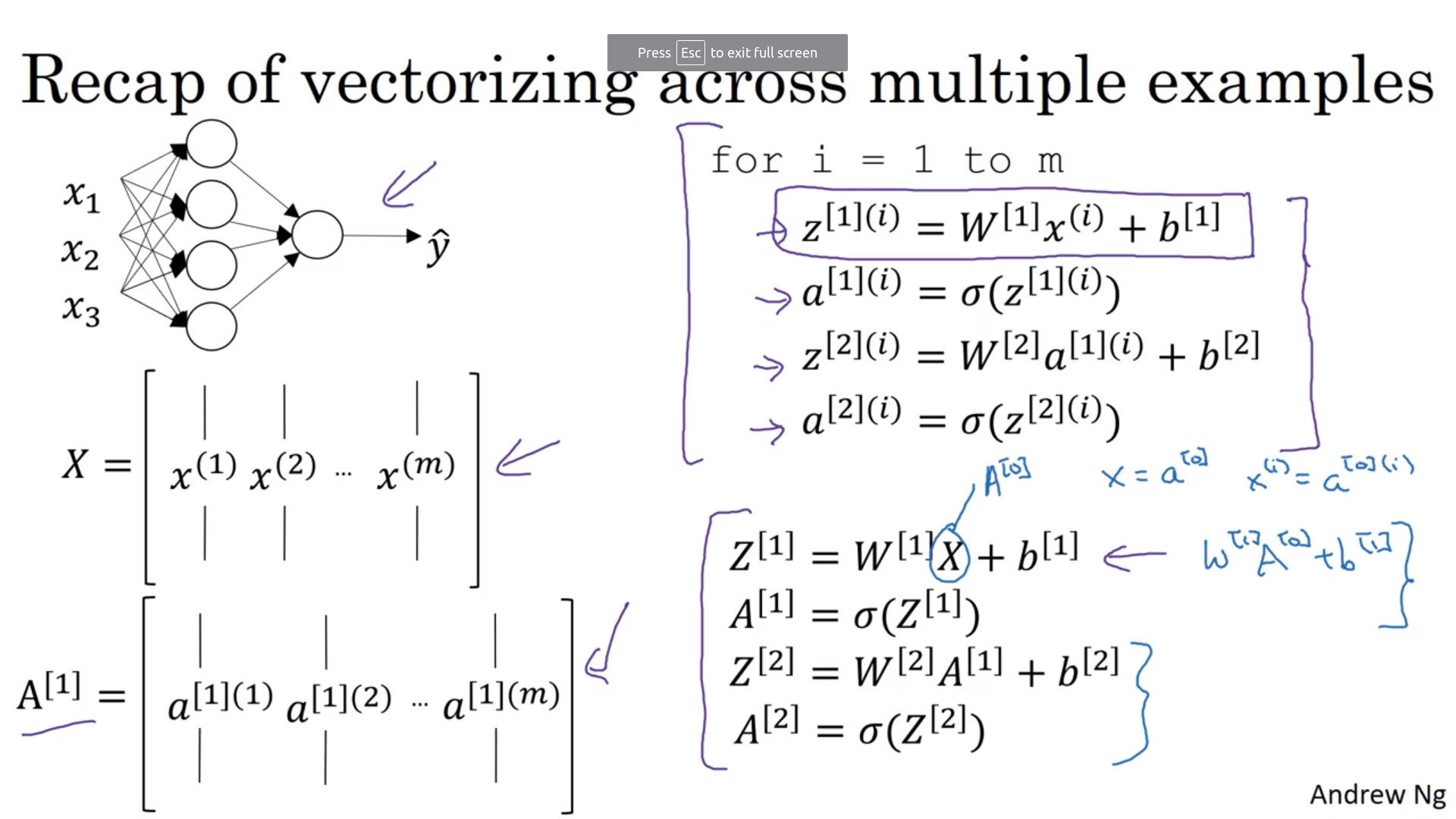#### 不同的activation function

sigmod函数的问题是它恒为正，所以算出来的结果不会关于0对称。所以隐藏层一般用$\tanh(z)$而不是sigmod，但是输出层还是用回sigmod。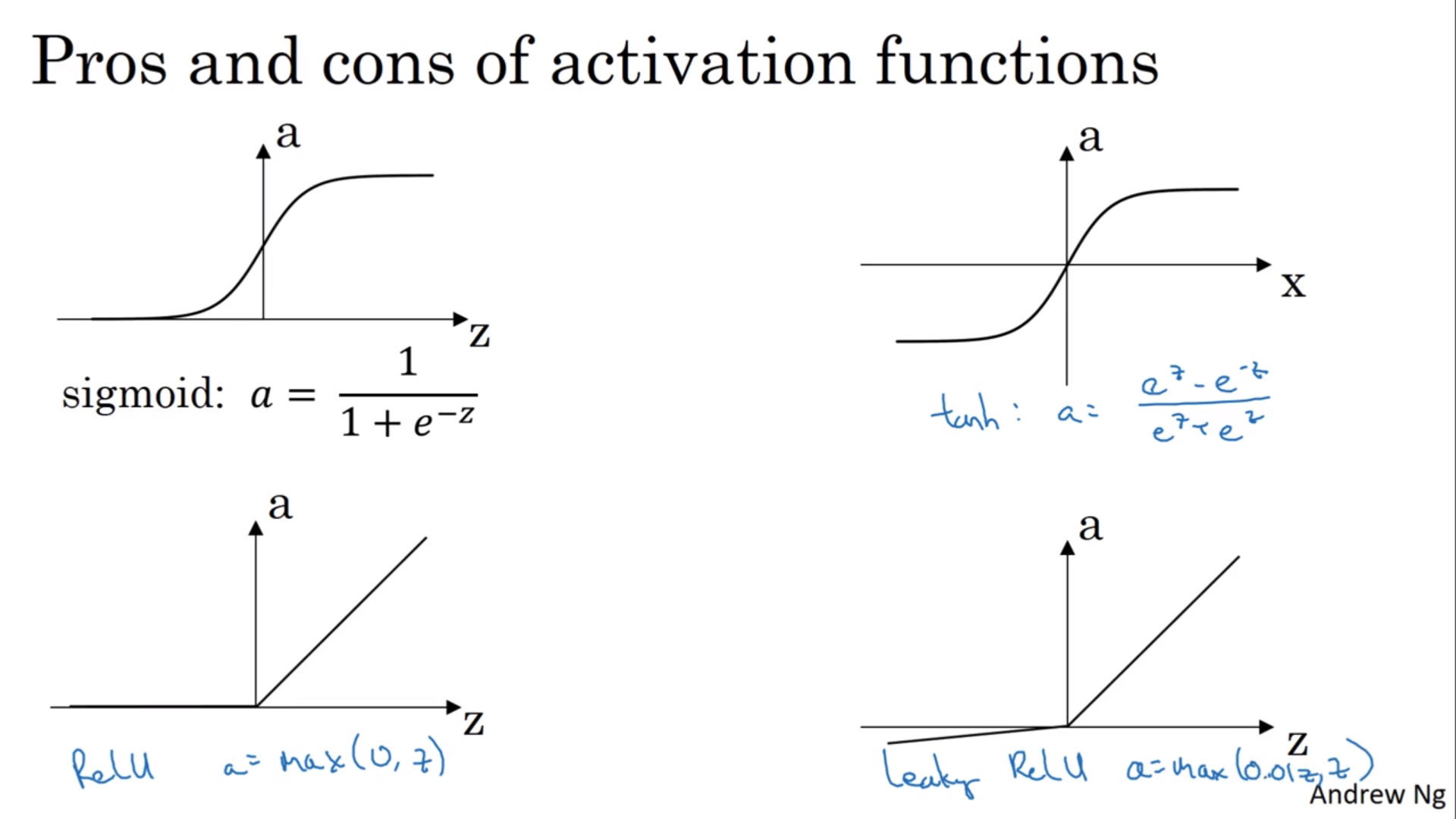#### 为什么activation function不是线性的？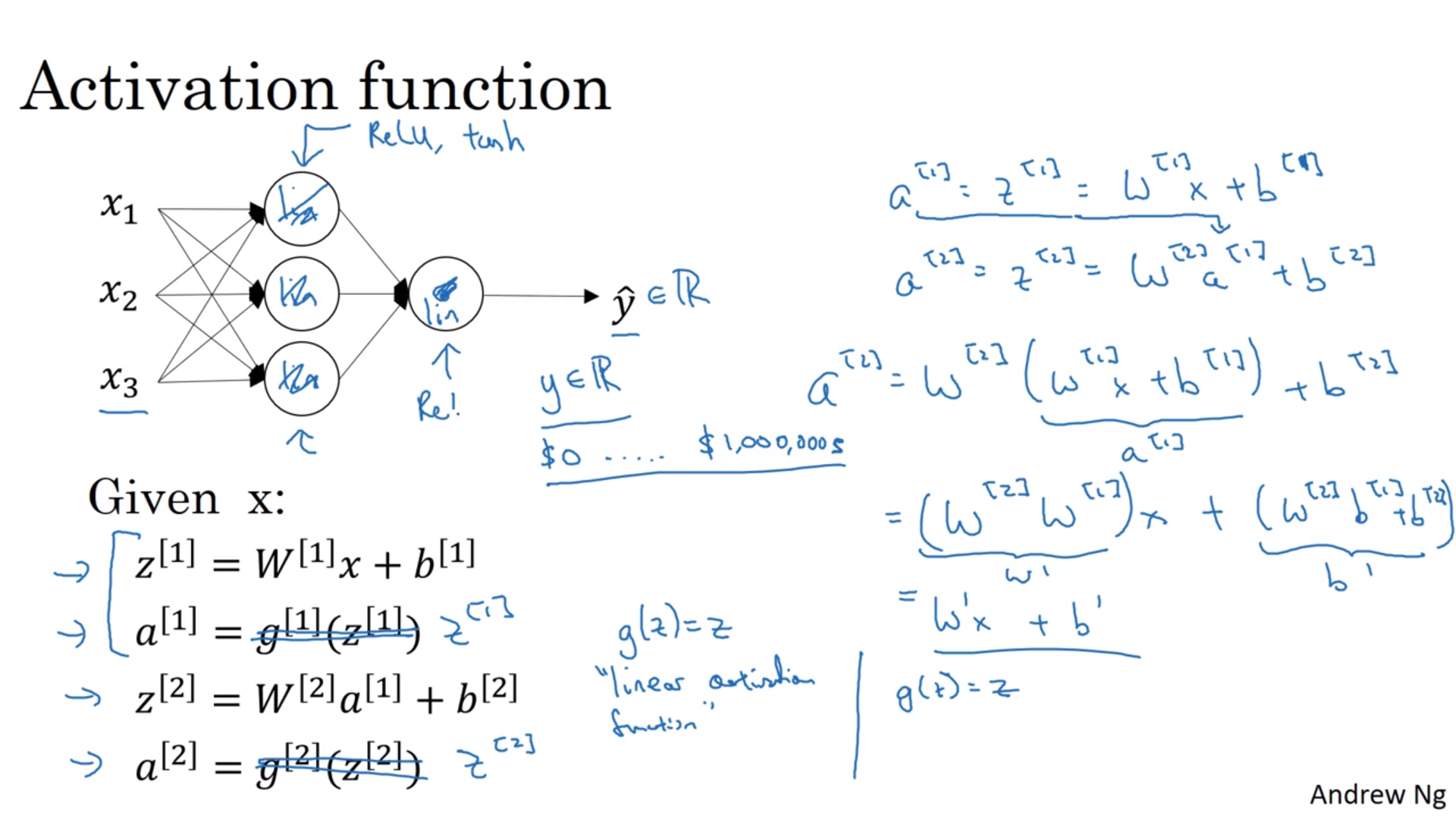#### AF的导数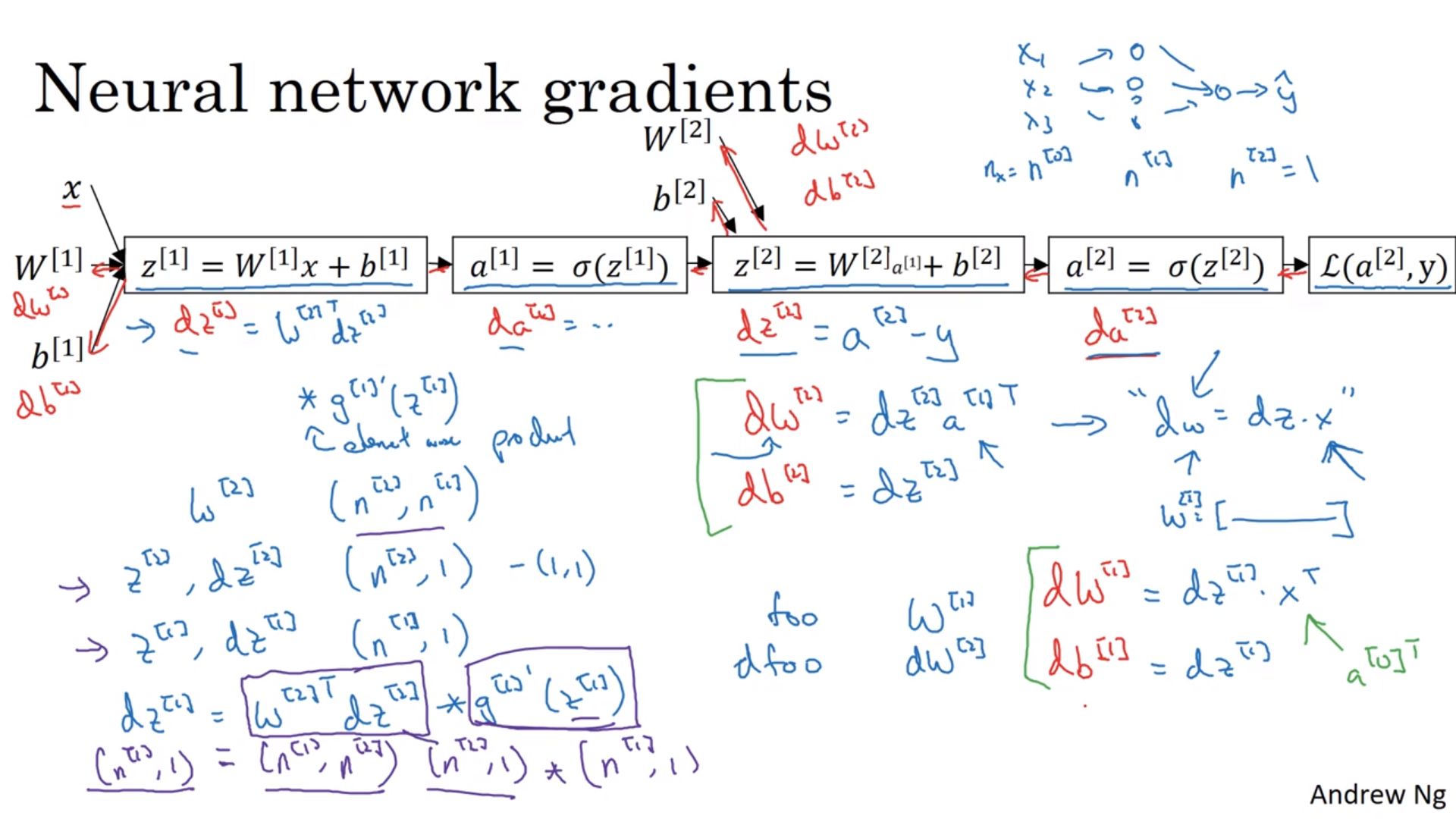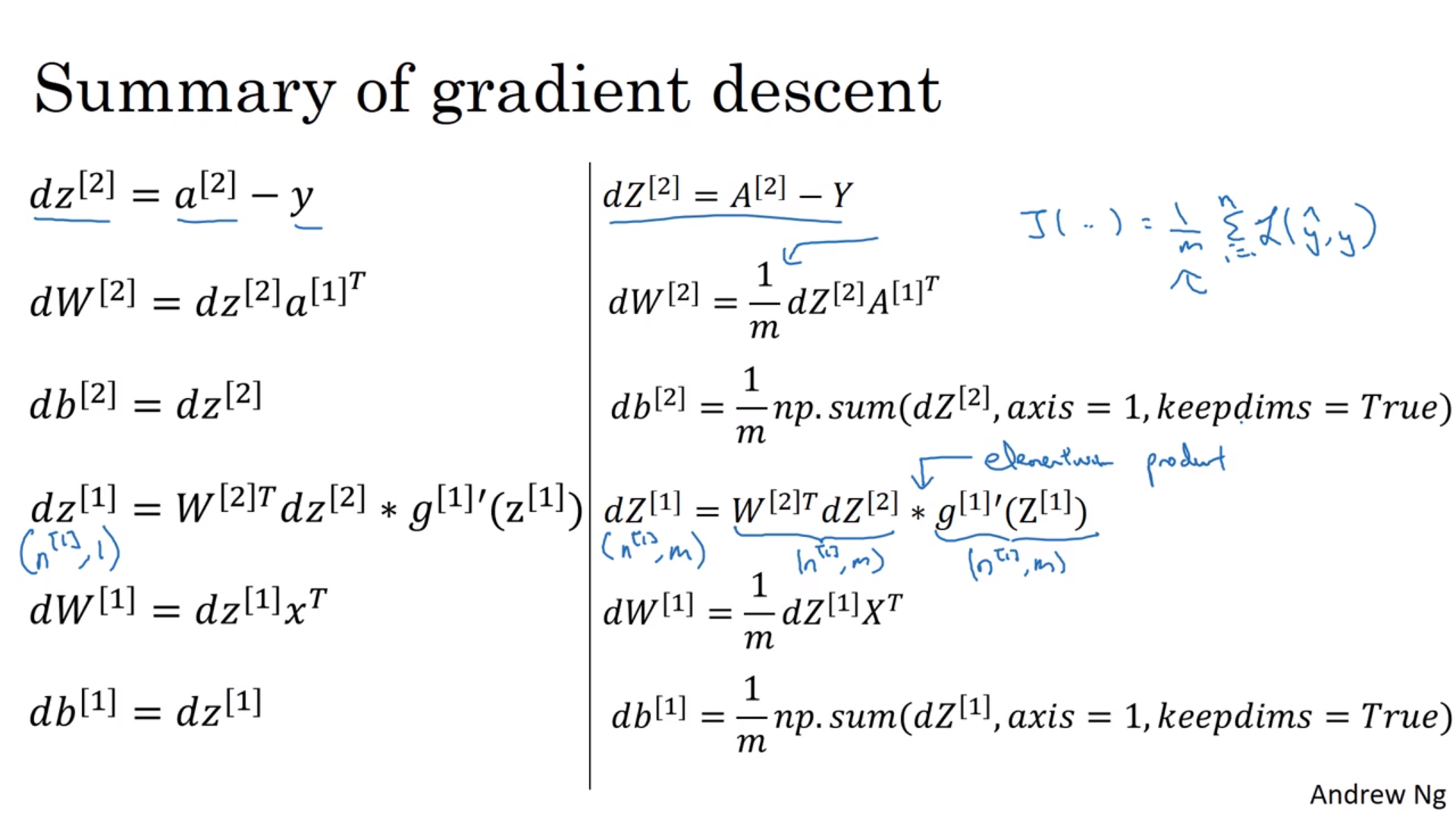#### 随机初值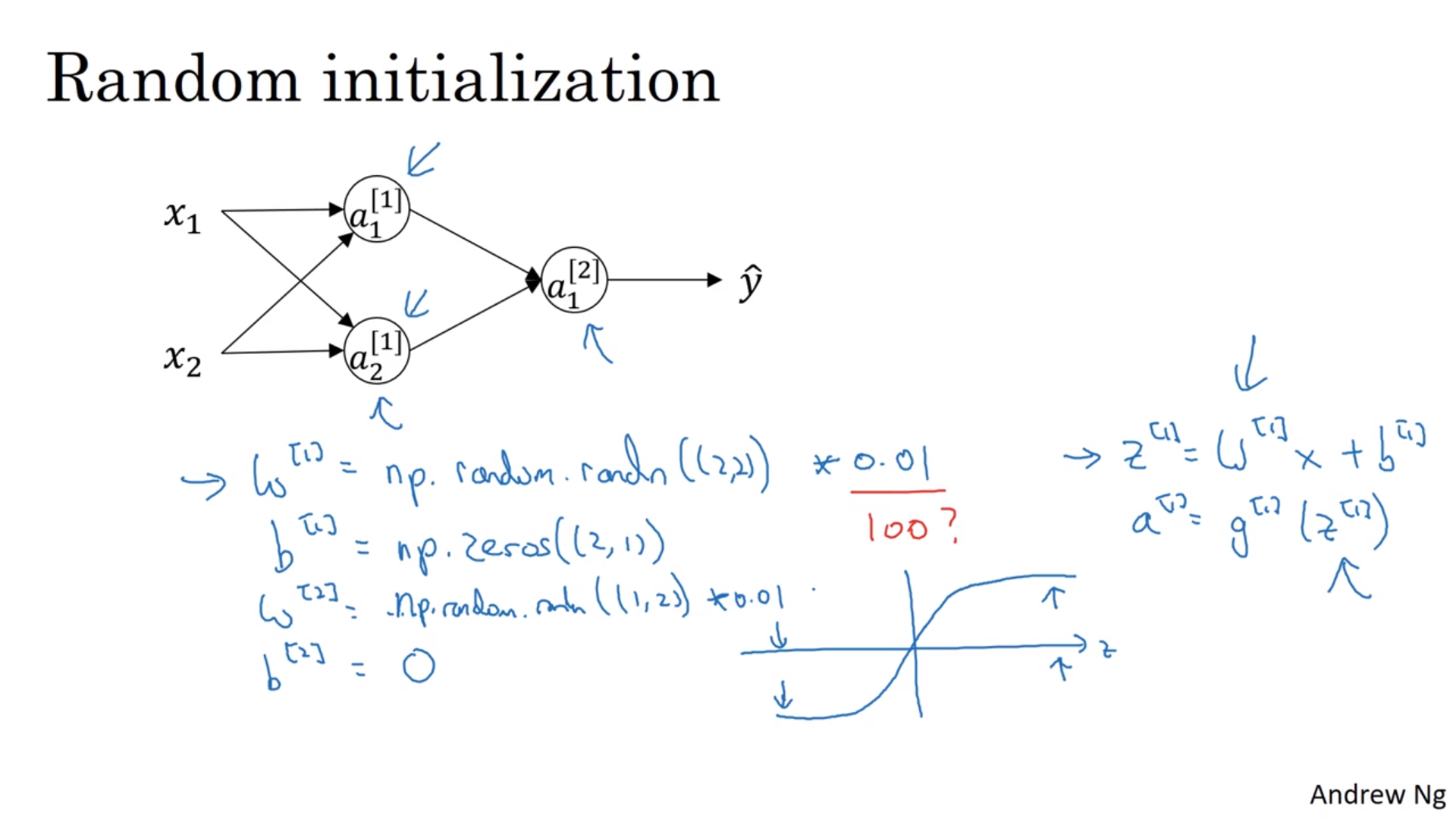### 深神经网络

#### 为什么要用深的神经网络？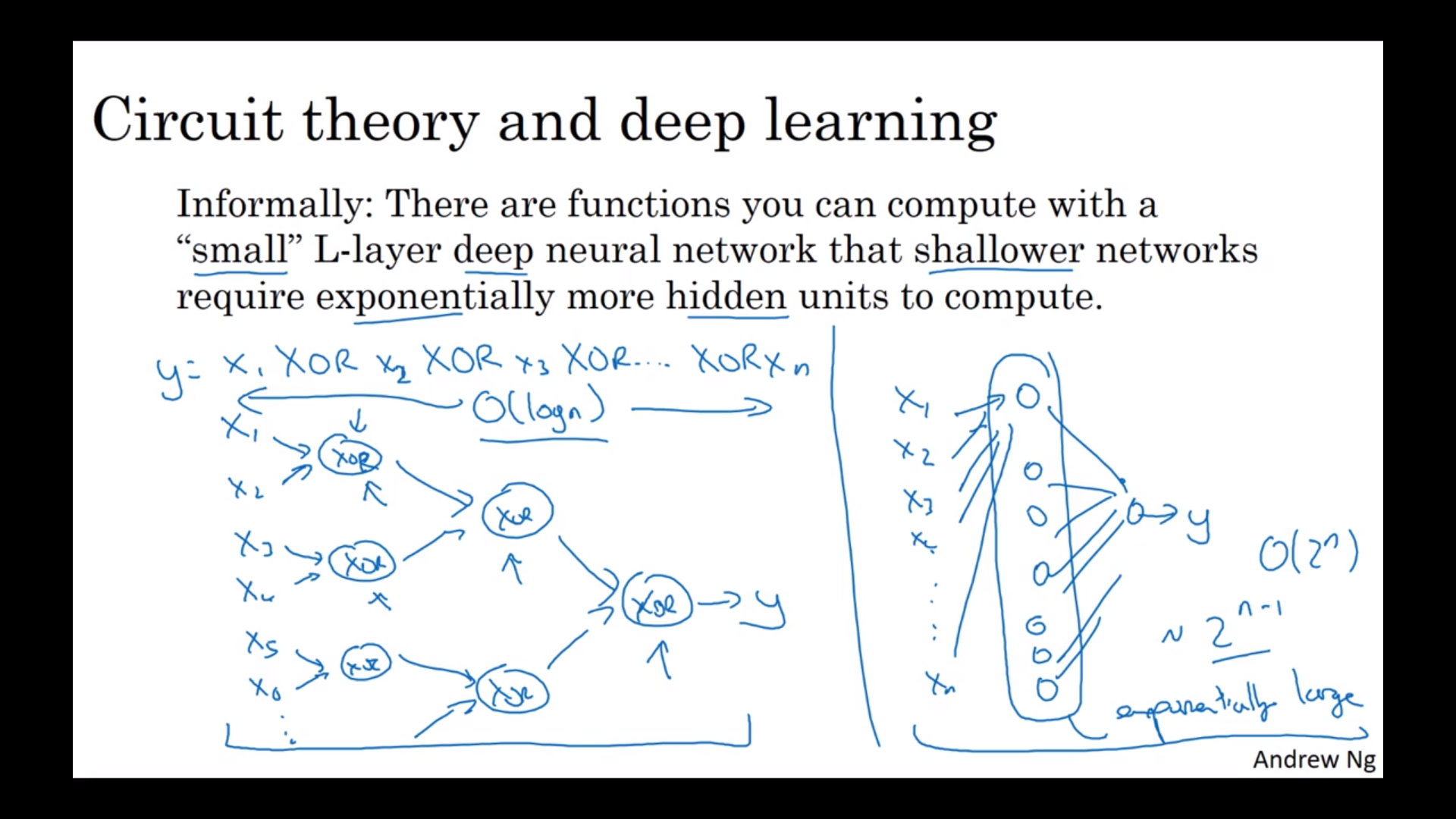#### 正向传递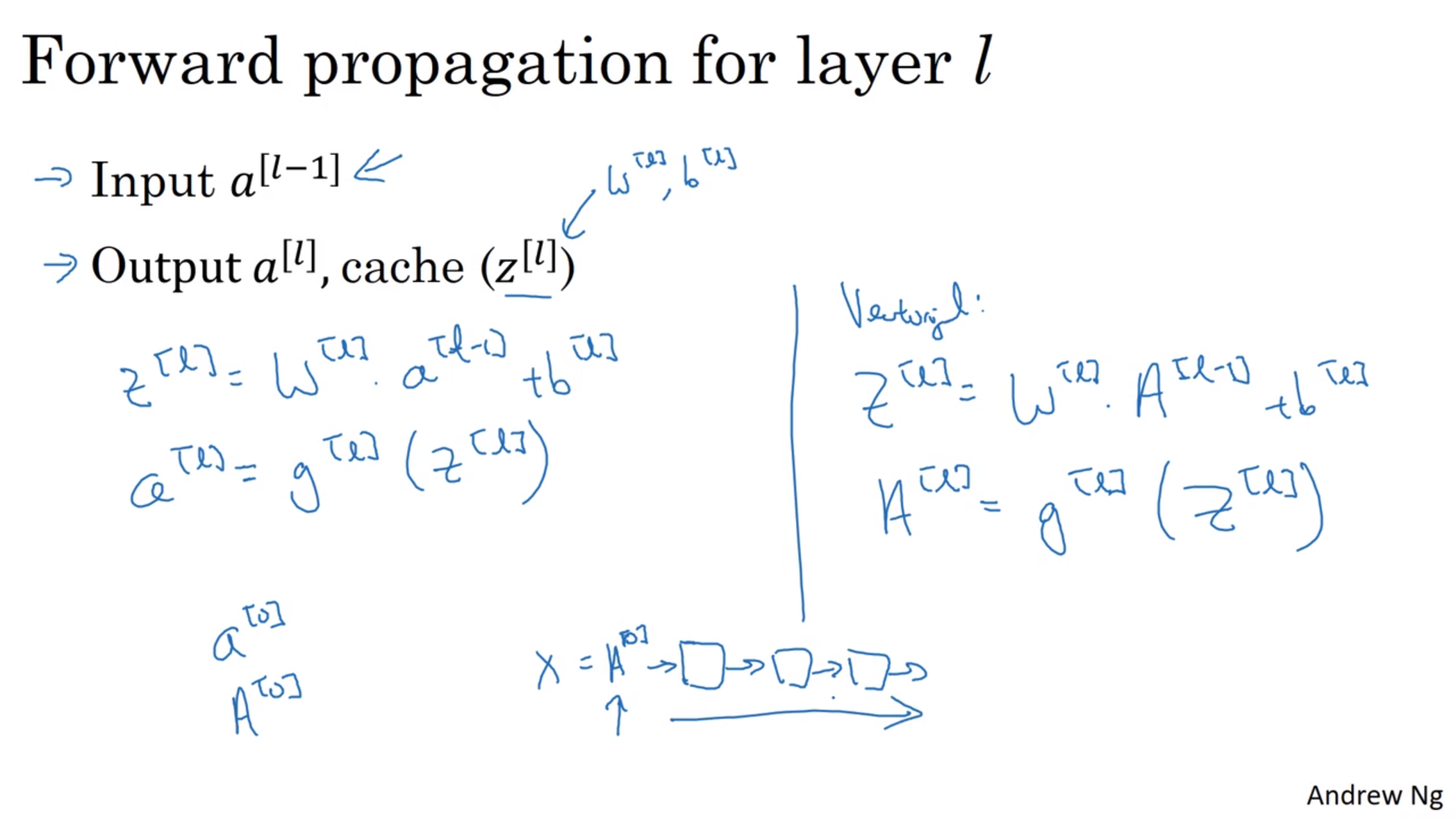#### 反向传递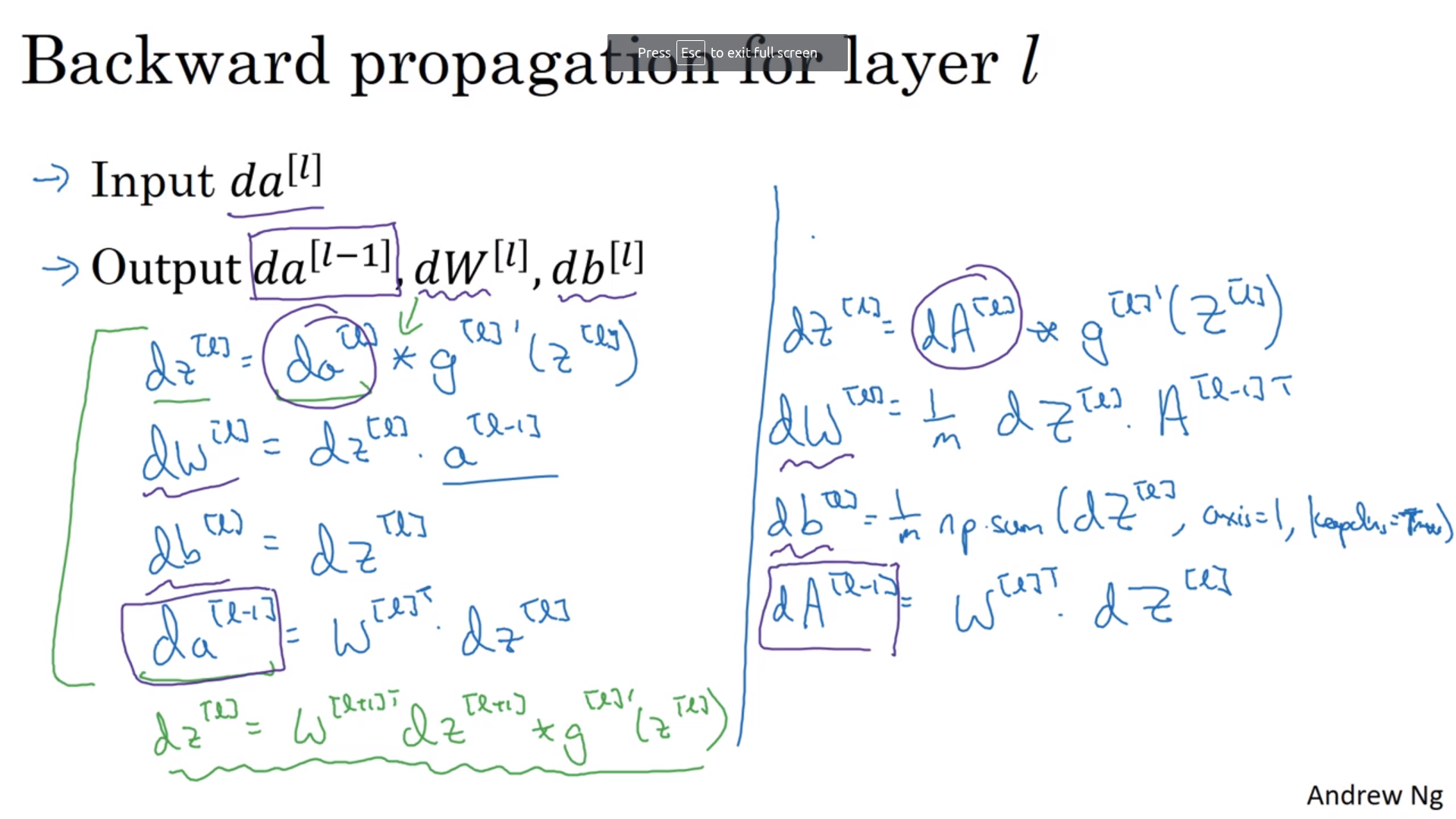#### 具体做法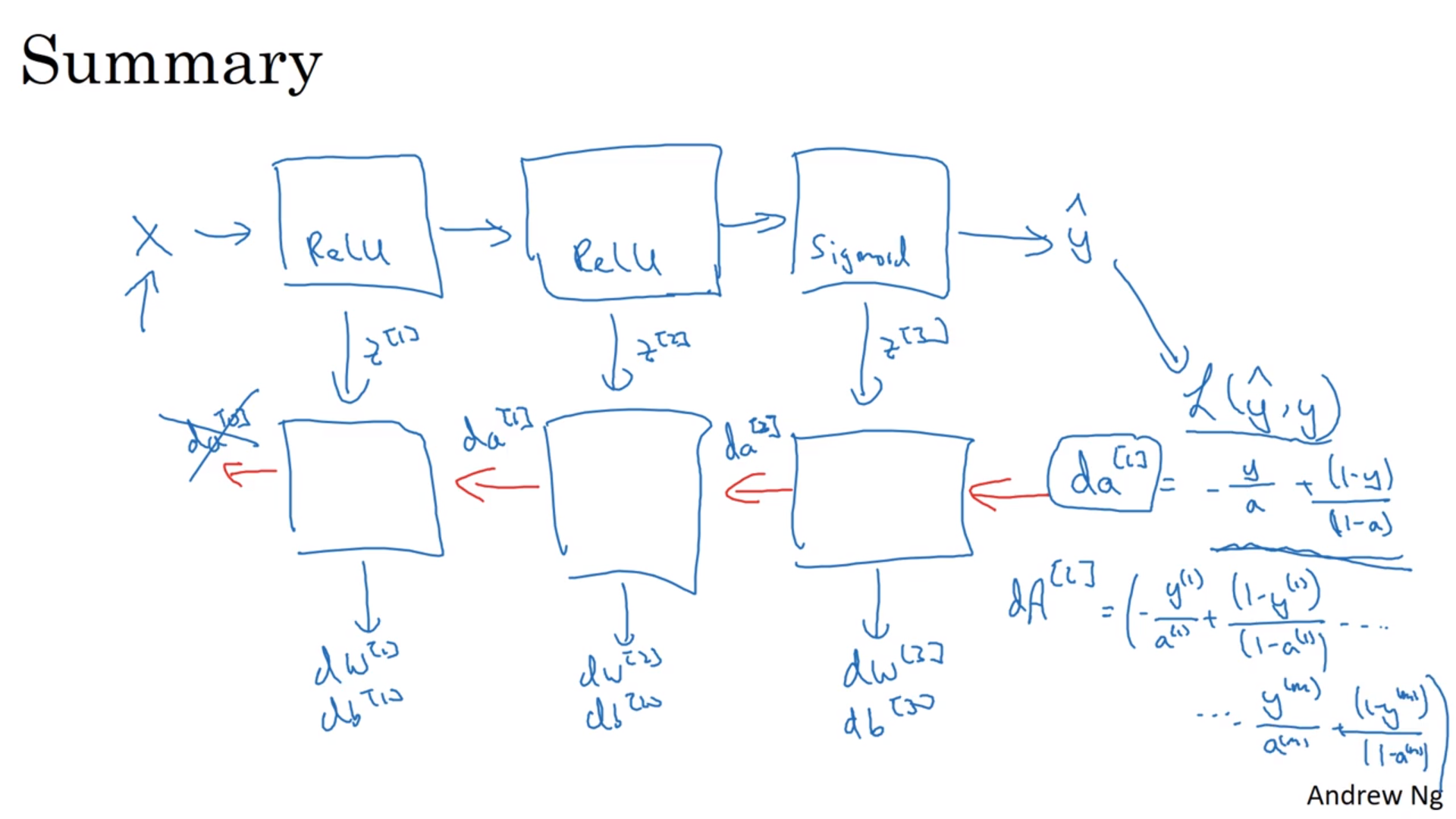#### 参数和超参数